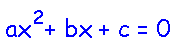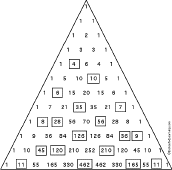More on Math Polynomials Quadratic Equations

A polynomial is a sum or difference of terms; each term is:
• a constant (like 5)
• a constant times a variable (like 3x)
• a constant times the variable to a positive integer power (like 2x2)
• a constant times the product of variables to positive integer powers (like 2x3y).
A monomial is a polynomial with only one term. A binomial is a polynomial that has two terms. A trinomial is a polynomial with three terms.

Degree of a Polynomial: The degree of a term within a polynomial is the sum of the exponents of variables that occur in that term (if there is no exponent written on a variable, such as in 3x, the exponent is one). The degree of a polynomial is the greatest degree of any term in the polynomial (for instance, for the polynomial 4x2 + 7xyz, the degree is 3 because of the last term).

• If a polynomial isn't just a constant and if each term has at most a variable to the first power (like 4x - 2 or 3y), then it is a first-degree polynomial (also called a linear polynomial).
• If a polynomial's highest degree is two, it is a quadratic (or second-degree) polynomial (example: 4x2 + 3x + 7).
• If a polynomial has more than one variable, then its degree is the sum of the exponents of the highest-degree term. For example, the polynomial 2xy2 -3xy + 6x - 2 has degree 3 (the sum of the xy2 exponents, 1 + 2).
Polynomials are often written in descending order, in which the terms with the largest powers are written first (like 9x2 - 3x + 6). If they are written with the smallest terms appearing first, this is ascending order (like 6 - 3x + 9x2).A polynomial equation is an equation involving a polynomial.

Multiplying Polynomials:
Multiply Polynomials: Introduction to Algebra - Worksheets to PrintMultiply Polynomials Worksheet #1Multiply 10 polynomials and put the answers in simplest form. Or go to the answers.Multiply Polynomials Worksheet #2Multiply 10 polynomials and put the answers in simplest form. Or go to the answers.

 Quadratic Equation: ax2 + bx - c = 0Printable Worksheets
MiscellaneousPascal's Triangle - WorksheetFill in the missing entries in Pascal's Triangle. Go to the answers.

Enchanted Learning®
Over 35,000 Web Pages
Sample Pages for Prospective Subscribers, or click below

 Overview of Site What's New Enchanted Learning Home Monthly Activity Calendar Books to Print Site Index K-3 Crafts K-3 Themes Little ExplorersPicture dictionary PreK/K Activities Rebus Rhymes Stories Writing Cloze Activities Essay Topics Newspaper Writing Activities Parts of Speech Fiction The Test of Time iPhone app TapQuiz Maps - free iPhone Geography Game Biology Animal Printouts Biology Label Printouts Biomes Birds Butterflies Dinosaurs Food Chain Human Anatomy Mammals Plants Rainforests Sharks Whales Physical Sciences: K-12 Astronomy The Earth Geology Hurricanes Landforms Oceans Tsunami Volcano Languages Dutch French German Italian Japanese (Romaji) Portuguese Spanish Swedish Geography/History Explorers Flags Geography Inventors US History Other Topics Art and Artists Calendars College Finder Crafts Graphic Organizers Label Me! Printouts Math Music Word Wheels

## Enchanted Learning Search

 Search the Enchanted Learning website for: